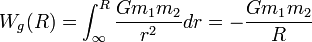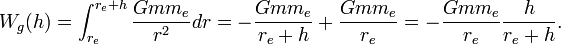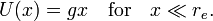# Gravitation

In physics, gravitation or gravity is the tendency of objects with mass to accelerate toward each other, or, in other words, two masses attract each other. Gravitation is one of the four fundamental interactions in nature, the other three being the electromagnetic force, the weak nuclear force, and the strong nuclear force. Gravitation is the weakest of these interactions, but acts over great distances and is always attractive. In classical mechanics, gravitation is given by Newton's gravitational force, which is an inverse-square law. In general relativity, gravitation arises out of spacetime being curved by the presence of mass, and is not a force. In quantum gravity theories, either the graviton is the postulated carrier of the gravitational force, or time-space itself is envisioned as discrete in nature, or both.

Classically, the gravitational attraction of the earth endows objects with weight and causes them to fall to the ground when dropped. Moreover, gravitation is the reason for the very existence of the earth, the sun, and other celestial bodies; without it matter would not have coalesced into these bodies and life as we know it would not exist. Gravitation is also responsible for keeping the earth and the other planets in their orbits around the sun, the moon in its orbit around the earth, for the formation of tides, and for various other natural phenomena that we observe.

The acceleration due to gravity of a body in the vicinity of the earth is approximately 9.8 ms−2.

##  History of gravitational theory

Since the time of the Greek philosopher Aristotle in the 4th century BC, there have been many attempts to understand and explain gravity. Aristotle believed that there was no effect without a cause, and therefore no motion without a force. He hypothesized that everything tried to move towards their proper place in the crystalline spheres of the heavens, and that physical bodies fell toward the center of the Earth in proportion to their weight. Another example of an attempted explanation is that of the Indian astronomer Brahmagupta who, in 628 AD, wrote that "bodies fall towards the earth as it is in the nature of the earth to attract bodies, just as it is in the nature of water to flow".

In 1687, the English physicist and mathematician Sir Isaac Newton published the famous Principia, which presented the inverse-square law of universal gravitation. In his own words he described the ideas that came to him in 1665, as a twenty-two year old student:

And the same year I began to think of gravity extending to ye orb of the Moon & [...] from Keplers rule of the periodical times of the Planets being in sesquialterate proportion of their distances from the center of their Orbs, I deduced that the forces wch keep the Planets in their Orbs must [be] reciprocally as the squares of their distances from the centers about wch they revolve; & thereby compared the force requisite to keep the Moon in her Orb with the force of gravity at the surface of the earth, & found them answer pretty nearly.

Most modern non-relativistic gravitational calculations are based on Newton's work.

Between 1909 and 1915 Albert Einstein worked on his general theory of relativity, which furnishes a deep understanding of gravitation. Einstein's work culminated in the final form of his gravitational equations, presented on November 25, 1915.

## Newton's law of universal gravitation

In 1687 Newton published his work on the universal law of gravity in his book Philosophiae Naturalis Principia Mathematica (Mathematical Principles of Natural Philosophy). Newton’s law of gravitation states that: every particle in the universe attracts every other particle with a force that is directly proportional to the product of their masses and inversely proportional to the square of the distance between them. If the particles have masses m1 and m2 and are separated by a distance r (from their centers of gravity), the magnitude of this gravitational force is:$F = G \frac{m_1 m_2}{r^2}$

where:

F is the magnitude of the gravitational force between the two point masses
G is the universal gravitational constant, G = 6.673 84 × 10−11 m3kg−1s−2
m1 is the mass of the first point mass
m2 is the mass of the second point mass
r is the distance between the two point masses

## Gravitational potential

The above equation leads to the equation for the work done in moving a mass from infinity to a distance R, which is obtained by integrating the force of gravity:$W_g(R) = \int_{\infty}^{R} {G m_1 m_2 \over r^2} dr = - {G m_1 m_2 \over R}$

where G is the universal gravitational constant. The function Wg(R) is known as the gravitational potential energy of the two masses. Note that this potential energy is zero at infinity.

Similarly the work done in moving an object of mass m from the Earth's surface to a distance h above the surface is given by:$W_g(h) = \int_{r_e}^{r_e+h} {G m m_e \over r^2} dr = -\frac{Gm m_e}{r_e+h} +{G m m_e \over r_e} = -\frac{Gm m_e}{r_e} \frac{h}{r_e+h} .$

Here re is the Earth's radius, and me is the Earth's mass. The classical gravitational field is,$U(h) = g h \frac{r_e}{r_e+h} \quad\hbox{with}\quad g \equiv \frac{G m_e}{r_e^2},$

where the value of the gravitational acceleration g = 9.81 m/s2 on earth. The zero of the potential U(h) is for h = 0 (the surface of the earth). Note that for small heights hx << re the gravitational potential field is linear in the height,$U(x) = g x \quad\hbox{for}\quad x \ll r_e.$

The corresponding force on a particle of mass m is (valid for small heights),$F = - m \frac{d U(x)}{dx} = -mg = ma\quad\hbox{with}\quad a\equiv \frac{d^2 x}{dt^2}.$

Integration, while using that the speed v of the mass at initial time is zero, gives$v(t) = -gt \quad \hbox{and}\quad x(t) = -\frac{1}{2} g x t^2 + x_0.$

This means that, ignoring air resistance, an object falling freely near the Earth's surface increases in speed by 9.81 m/s (around 22 mph) for each second of its descent. Thus, an object starting from rest will attain a speed of 9.81 m/s after one second, 19.62 m/s after two seconds, and so on.

## General relativity

Newton's conception and quantification of gravitation held until the beginning of the 20th century, when the German-born physicist Albert Einstein proposed the general theory of relativity. In this theory Einstein proposed that inertial motion occurs when objects are in free-fall instead of when they are at rest with respect to a massive object such as the Earth (as is the case in classical mechanics). The problem is that in flat spacetimes such as those of classical mechanics and special relativity, there is no way that inertial observers can accelerate with respect to each other, as free-falling bodies can do as they each are accelerated towards the center of a massive object.

To deal with this difficulty, Einstein proposed that spacetime is curved by the presence of matter, and that free-falling objects are following the geodesics of the spacetime. More specifically, Einstein discovered the field equations of general relativity, which relate the presence of matter and the curvature of spacetime. The Einstein field equations are a set of 10 simultaneous, non-linear, differential equations whose solutions give the components of the metric tensor of spacetime. This metric tensor allows to calculate not only angles and distances between space-time intervals (segments) measured with the coordinates against which the spacetime manifold is being mapped but also the affine-connection from which the curvature is obtained, thereby describing the spacetime's geometrical structure. Notable solutions of the Einstein field equations include:

General relativity has enjoyed much success because of how its predictions have been regularly confirmed. For example:

## Alternative theories

Historical alternative theories

Recent alternative theories